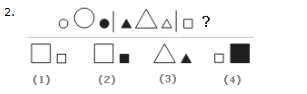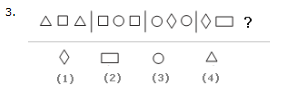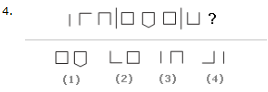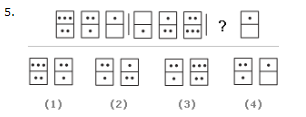Direction Look carefully at the sequence of symbols to find the pattern. Select correct pattern.
1.A. 1 B. 2 C. 3 D. 4
Explanation:

Explanation: This is an alternating series. In the first segment, the letter "E" faces right, then down, then right. In the second segment, the letters all face down. To follow this pattern, in the fourth segment, the letters must all face up.

2.A. 1 B. 2 C. 3 D. 4
Explanation:

Explanation: Notice that in each segment, the figures are all the same shape, but the one in the middle is larger than the two on either side. Also, notice that one of the figures is shaded and that this shading alternates first right and then left. To continue this pattern in the third segment, you will look for a square. Choice b is correct because this choice will put the large square between the two smaller squares, with the shading on the right.

3.A. 1 B. 2 C. 3 D. 4
Explanation:

Explanation: Look at each segment. You will notice that in each, the figure on the right and the figure on the left are the same; the figure in between is different. To continue this pattern in the last segment, the diamond on the left will be repeated on the right. Choice a is the only possible answer.

4.A. 1 B. 2 C. 3 D. 4
Explanation:

Explanation: This sequence concerns the number of sides on each figure. In the first segment, the three figures have one side, and then two sides, and then three sides. In the second segment, the number of sides increases and then decreases. In the third segment, the number of sides continues to decrease.

5.A. 1 B. 2 C. 3 D. 4